# Graphs Graphs Applications of DepthFirst Search Undirected graphs

• Slides: 52
Download presentation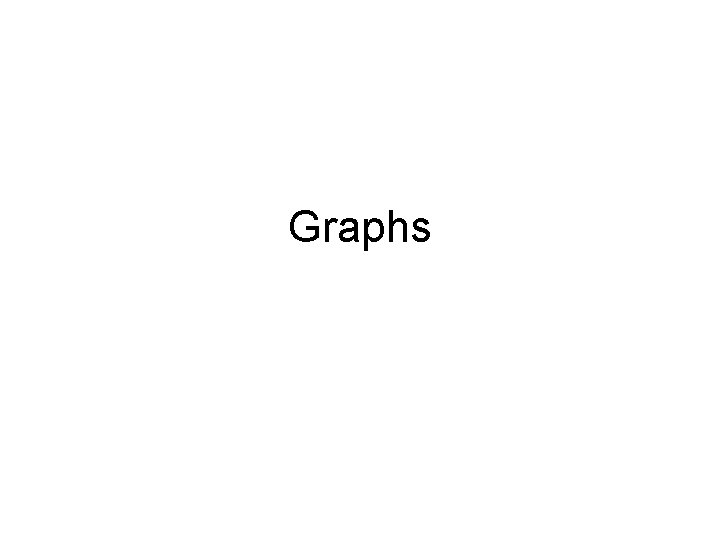GraphsGraphs • Applications of Depth-First Search – Undirected graphs: • Connected components, articulation points, bridges, biconnected components – Directed graphs: • Cyclic/acyclic graphs • Topological sort • Strongly connected componentsConnectivity, connected components • An undirected graph is called a connected graph if there is a path between any two vertices. • A connected component of an undirected graph is a subgraph in which any two vertices are connected to each other by paths, and which is connected to no additional vertices in the supergraph.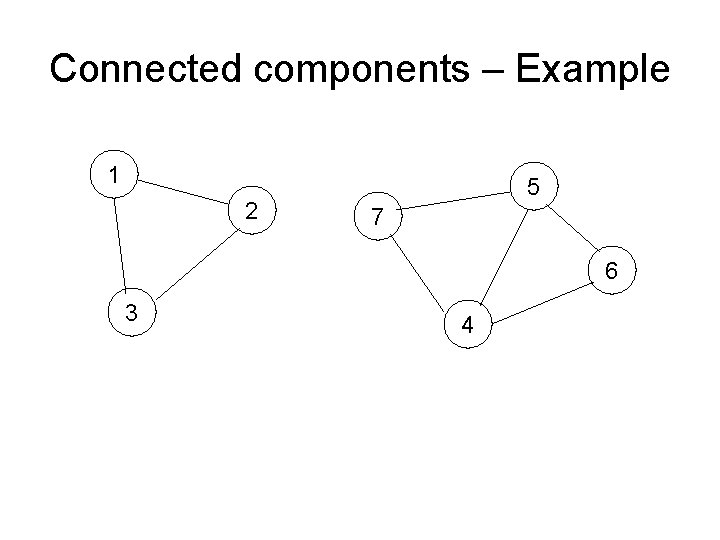Connected components – Example 1 2 5 7 6 3 4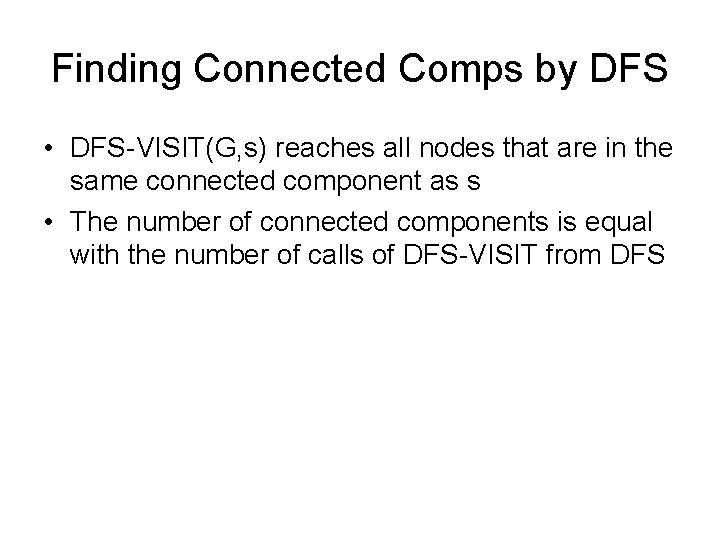Finding Connected Comps by DFS • DFS-VISIT(G, s) reaches all nodes that are in the same connected component as s • The number of connected components is equal with the number of calls of DFS-VISIT from DFS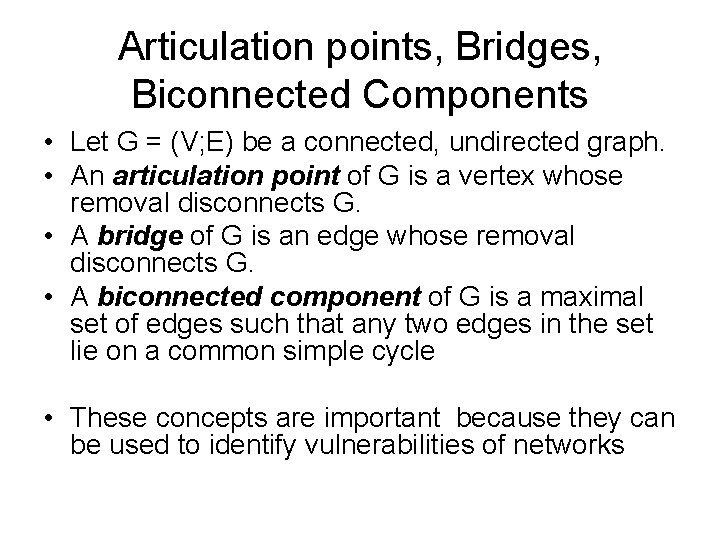Articulation points, Bridges, Biconnected Components • Let G = (V; E) be a connected, undirected graph. • An articulation point of G is a vertex whose removal disconnects G. • A bridge of G is an edge whose removal disconnects G. • A biconnected component of G is a maximal set of edges such that any two edges in the set lie on a common simple cycle • These concepts are important because they can be used to identify vulnerabilities of networks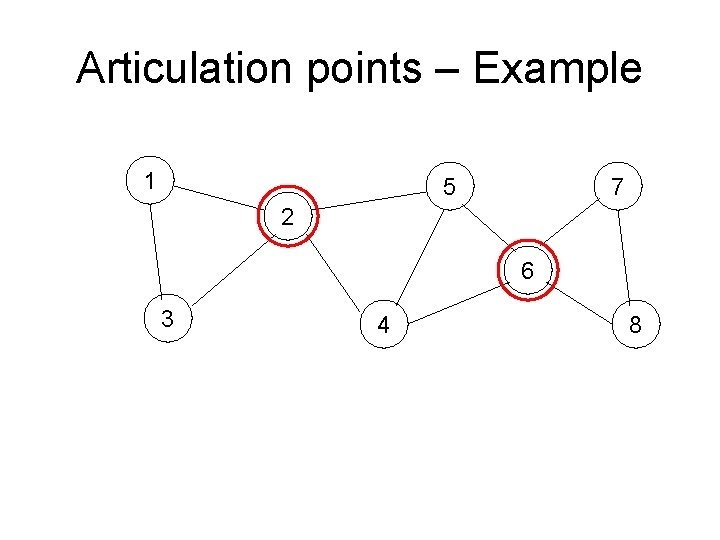Articulation points – Example 1 5 7 2 6 3 4 8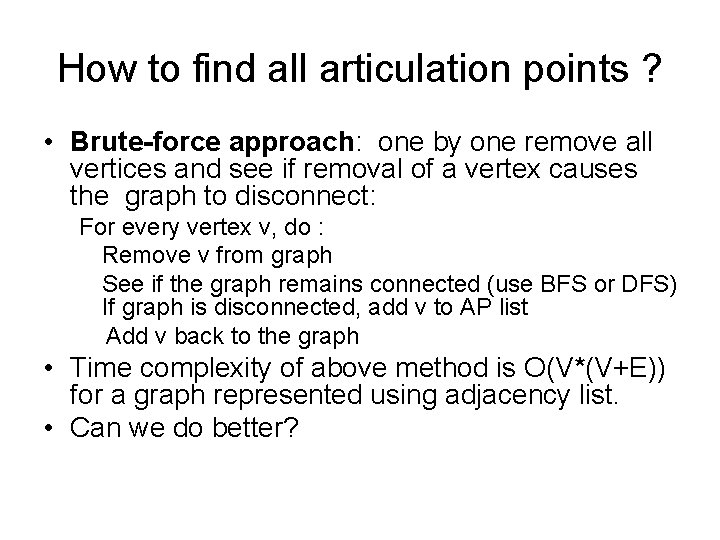How to find all articulation points ? • Brute-force approach: one by one remove all vertices and see if removal of a vertex causes the graph to disconnect: For every vertex v, do : Remove v from graph See if the graph remains connected (use BFS or DFS) If graph is disconnected, add v to AP list Add v back to the graph • Time complexity of above method is O(V*(V+E)) for a graph represented using adjacency list. • Can we do better?How to find all articulation points ? • • DFS- based-approach: We can prove following properties: 1. The root of a DFS-tree is an articulation point if and only if it has at least two children. 2. A nonroot vertex v of a DFS-tree is an articulation point of G if and only if has a child s such that there is no back edge from s or any descendant of s to a proper ancestor of v. 3. Leafs of a DFS-tree are never articulation points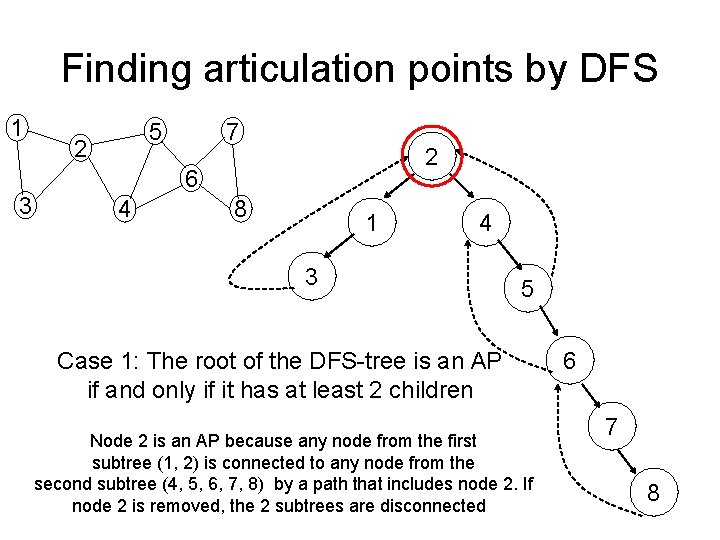Finding articulation points by DFS 1 5 2 7 2 6 3 4 8 1 4 3 5 Case 1: The root of the DFS-tree is an AP if and only if it has at least 2 children Node 2 is an AP because any node from the first subtree (1, 2) is connected to any node from the second subtree (4, 5, 6, 7, 8) by a path that includes node 2. If node 2 is removed, the 2 subtrees are disconnected 6 7 8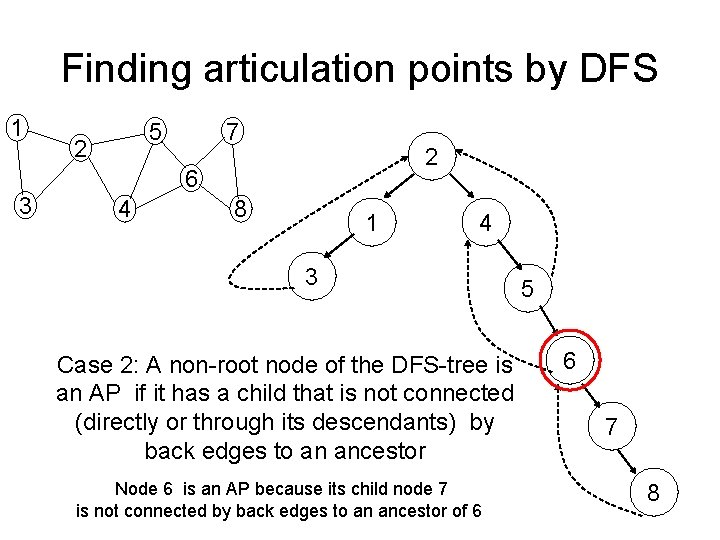Finding articulation points by DFS 1 5 2 7 2 6 3 4 8 1 4 3 Case 2: A non-root node of the DFS-tree is an AP if it has a child that is not connected (directly or through its descendants) by back edges to an ancestor Node 6 is an AP because its child node 7 is not connected by back edges to an ancestor of 6 5 6 7 8Finding articulation points by DFS 1 5 2 7 2 6 3 4 8 1 4 3 5 6 Case 2: A non-root node of the DFS-tree is an AP if it has a child that is not connected (directly or through its descendants) by 7 back edges to an ancestor How can we efficiently implement the test for this case ? Node 6 is an AP because its child node 7 is not connected by back edges to an ancestor of 6 The LOW function ! 8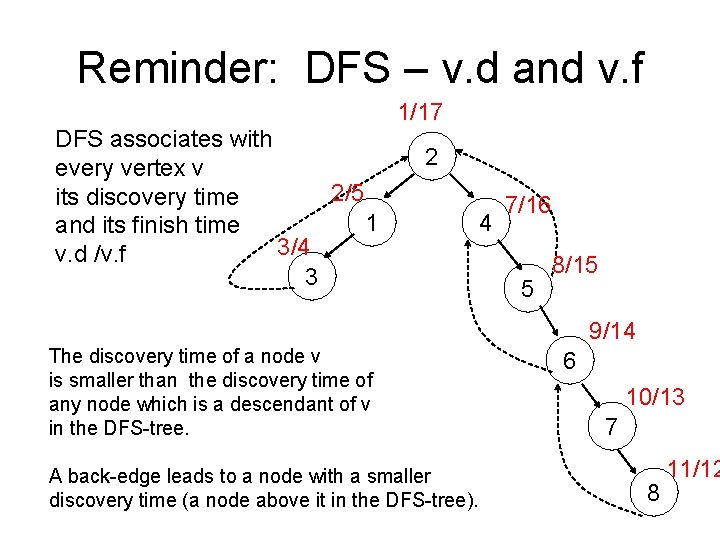Reminder: DFS – v. d and v. f 1/17 DFS associates with every vertex v 2/5 its discovery time 1 and its finish time 3/4 v. d /v. f 3 2 4 7/16 5 8/15 9/14 The discovery time of a node v is smaller than the discovery time of any node which is a descendant of v in the DFS-tree. A back-edge leads to a node with a smaller discovery time (a node above it in the DFS-tree). 6 10/13 7 8 11/12The LOW function 1/17 Low=1 2/5 Low=1 1 3/4 Low=1 3 2 7/16 4 Low=1 8/15 5 Low=7 9/14 The LOW function: 6 Low=9 LOW(u) = the highest ancestor (identified by its 10/13 smallest discovery time) of u that can be reached 7 Low=9 from a descendant of u by using back-edges 11/12 u is articulation point if it has a descendant 8 v with LOW(v)>=u. d Low=9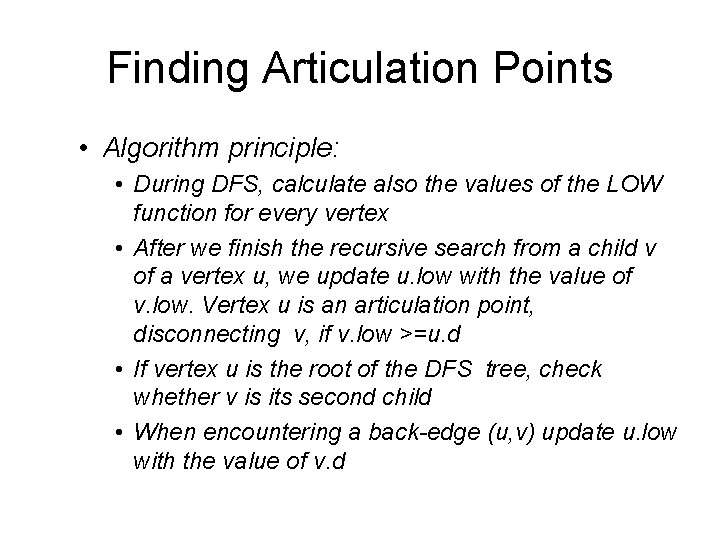Finding Articulation Points • Algorithm principle: • During DFS, calculate also the values of the LOW function for every vertex • After we finish the recursive search from a child v of a vertex u, we update u. low with the value of v. low. Vertex u is an articulation point, disconnecting v, if v. low >=u. d • If vertex u is the root of the DFS tree, check whether v is its second child • When encountering a back-edge (u, v) update u. low with the value of v. d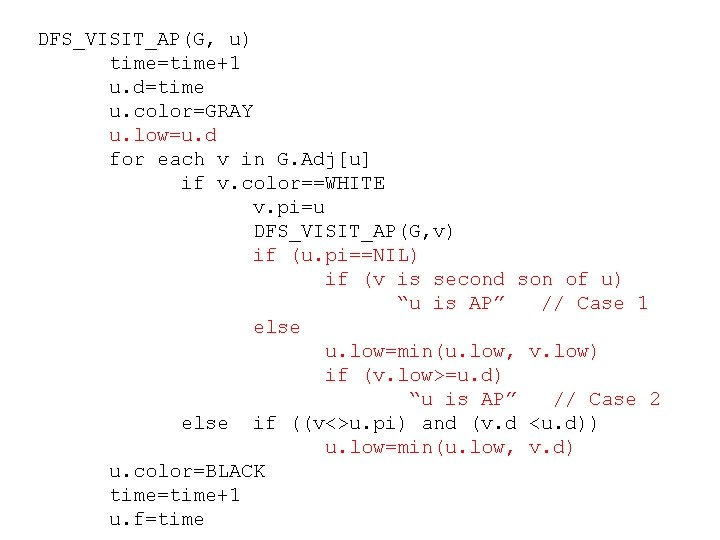DFS_VISIT_AP(G, u) time=time+1 u. d=time u. color=GRAY u. low=u. d for each v in G. Adj[u] if v. color==WHITE v. pi=u DFS_VISIT_AP(G, v) if (u. pi==NIL) if (v is second son of u) “u is AP” // Case 1 else u. low=min(u. low, v. low) if (v. low>=u. d) “u is AP” // Case 2 else if ((v<>u. pi) and (v. d <u. d)) u. low=min(u. low, v. d) u. color=BLACK time=time+1 u. f=timeBridge edges – Example 1 5 2 6 3 4How to find all bridges ? • Brute-force approach: one by one remove all edges and see if removal of an edge causes the graph to disconnect: For every edge e, do : Remove e from graph See if the graph remains connected (use BFS or DFS) If graph is disconnected, add e to B list Add e back to the graph • Time complexity of above method is O(E*(V+E)) for a graph represented using adjacency list. • Can we do better?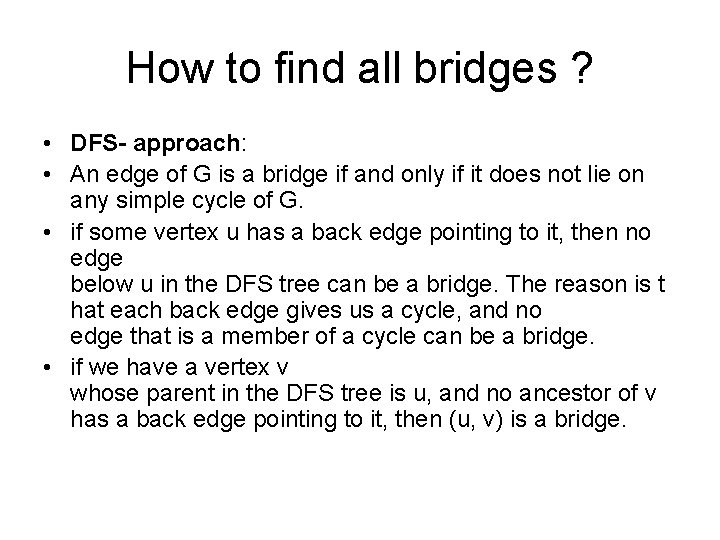How to find all bridges ? • DFS- approach: • An edge of G is a bridge if and only if it does not lie on any simple cycle of G. • if some vertex u has a back edge pointing to it, then no edge below u in the DFS tree can be a bridge. The reason is t hat each back edge gives us a cycle, and no edge that is a member of a cycle can be a bridge. • if we have a vertex v whose parent in the DFS tree is u, and no ancestor of v has a back edge pointing to it, then (u, v) is a bridge.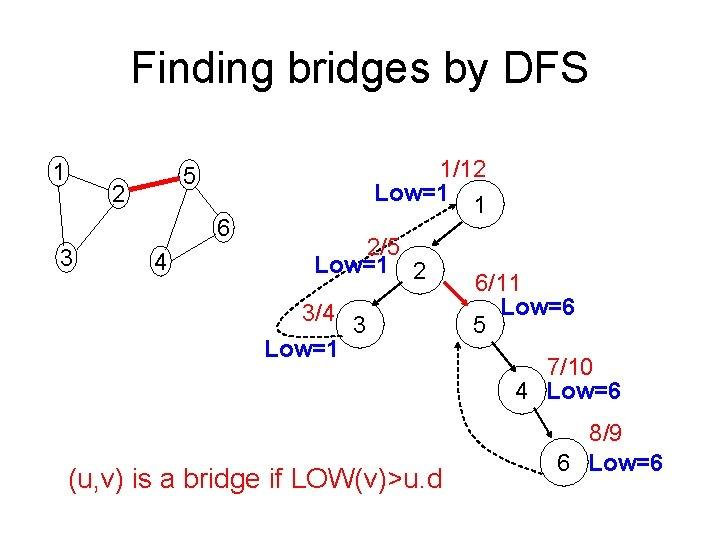Finding bridges by DFS 1 2 6 3 1/12 Low=1 1 5 4 2/5 Low=1 2 3/4 3 Low=1 (u, v) is a bridge if LOW(v)>u. d 6/11 Low=6 5 7/10 4 Low=6 8/9 6 Low=6DFS_VISIT_Bridges(G, u) time=time+1 u. d=time u. color=GRAY u. low=u. d for each v in G. Adj[u] if v. color==WHITE v. pi=u DFS_VISIT_AP(G, v) u. low=min(u. low, v. low) if (v. low>u. d) “(u, v) is Bridge” else if ((v<>u. pi) and (v. d <u. d)) u. low=min(u. low, v. d) u. color=BLACK time=time+1 u. f=timeBiconnected components – Example 1 5 2 6 3 4 7 8Finding biconnected components • Two biconnected components cannot have a common edge, but they can have a common vertex -> We will mark the edges with an id of their biconnected component • The common vertex of several biconnected components is an articulation point • The articulation points separate the biconnected components of a graph. If the graph has no articulation points, it is biconnex -> We will try to identify the biconnected components while searching for articulation pointsFinding biconnected components 1 2 3 5 4 6 7 8Finding biconnected components • Algorithm principle: • During DFS, use a stack to store visited edges (tree edges or back edges) • After we finish the recursive search from a child v of a vertex u, we check if u is an articulation point for v. If it is, we output all edges from the stack until (u, v). These edges form a biconnected component • When we return to the root of the DFS-tree, we have to output the edges even if the root is no articulation point (graph may be biconnex) – we will not test the case of the root being an articulation point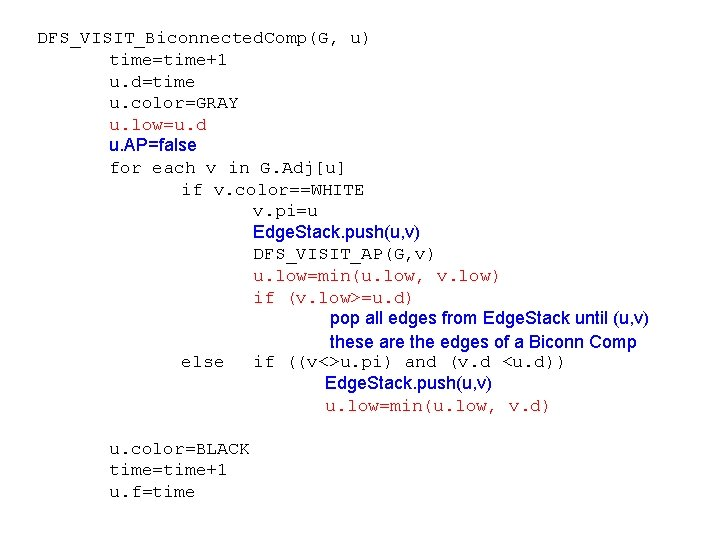DFS_VISIT_Biconnected. Comp(G, u) time=time+1 u. d=time u. color=GRAY u. low=u. d u. AP=false for each v in G. Adj[u] if v. color==WHITE v. pi=u Edge. Stack. push(u, v) DFS_VISIT_AP(G, v) u. low=min(u. low, v. low) if (v. low>=u. d) pop all edges from Edge. Stack until (u, v) these are the edges of a Biconn Comp else if ((v<>u. pi) and (v. d <u. d)) Edge. Stack. push(u, v) u. low=min(u. low, v. d) u. color=BLACK time=time+1 u. f=time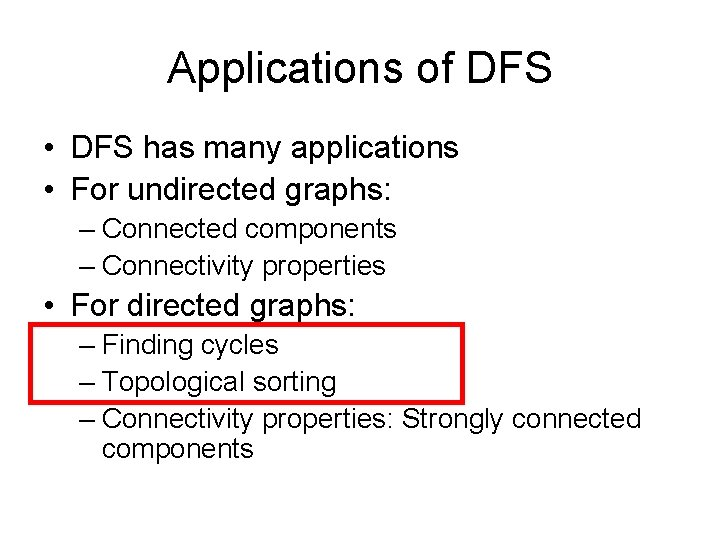Applications of DFS • DFS has many applications • For undirected graphs: – Connected components – Connectivity properties • For directed graphs: – Finding cycles – Topological sorting – Connectivity properties: Strongly connected components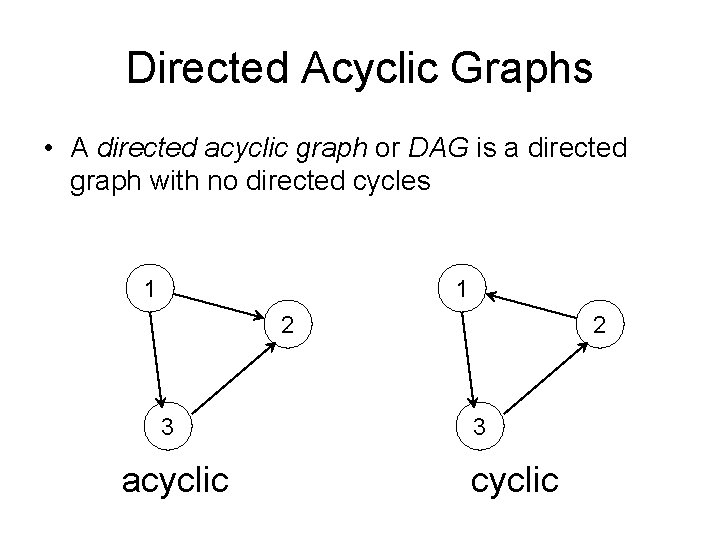Directed Acyclic Graphs • A directed acyclic graph or DAG is a directed graph with no directed cycles 1 1 2 3 acyclic 2 3 cyclic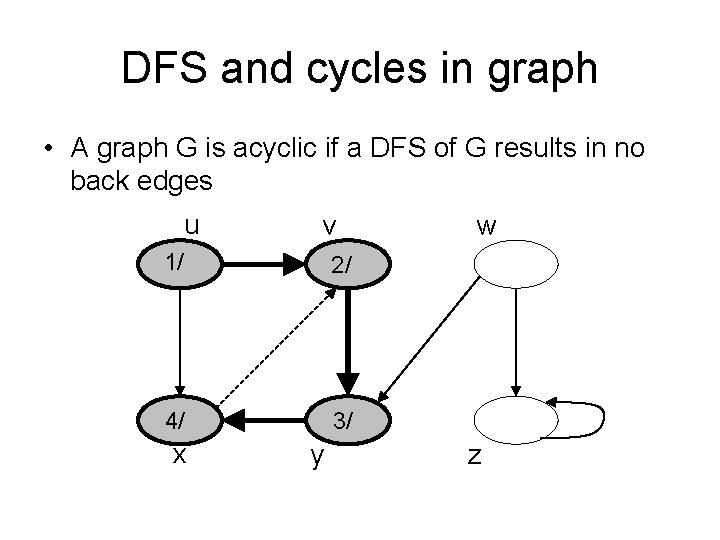DFS and cycles in graph • A graph G is acyclic if a DFS of G results in no back edges u v 1/ 2/ 4/ 3/ x y w z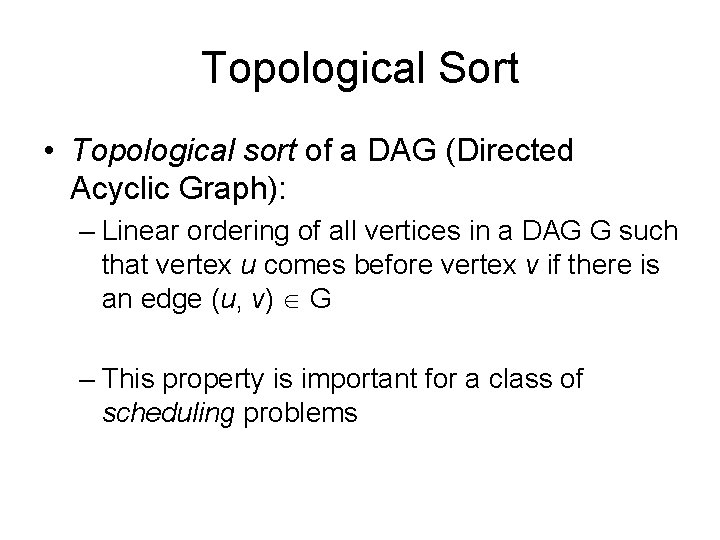Topological Sort • Topological sort of a DAG (Directed Acyclic Graph): – Linear ordering of all vertices in a DAG G such that vertex u comes before vertex v if there is an edge (u, v) G – This property is important for a class of scheduling problemsExample – Topological Sorting u v w x y z • There can be several orderings of the vertices that fulfill the topological sorting condition: – – u, v, w, y, x, z w, z, u, v, y, x w, u, v, y, x, z …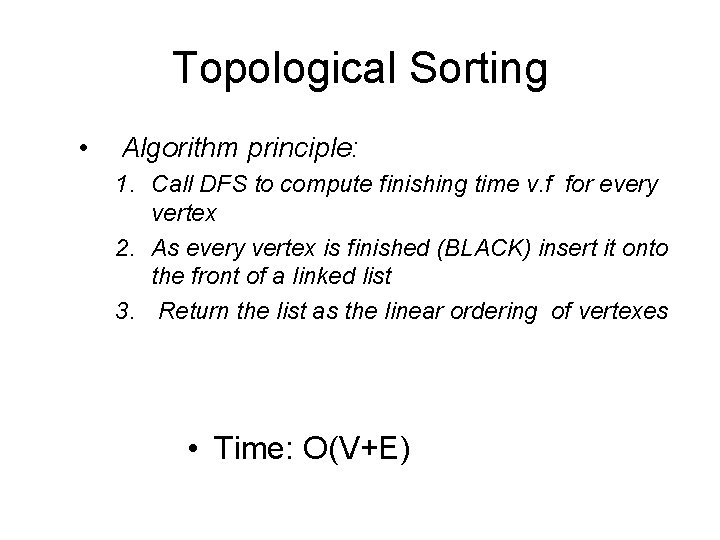Topological Sorting • Algorithm principle: 1. Call DFS to compute finishing time v. f for every vertex 2. As every vertex is finished (BLACK) insert it onto the front of a linked list 3. Return the list as the linear ordering of vertexes • Time: O(V+E)Using DFS for Topological SortingCorrectness of Topological Sort • Claim: (u, v) G u. f > v. f – When (u, v) is explored, u is grey • v = grey (u, v) is back edge. Contradiction, since G is DAG and contains no back edges • v = white v becomes descendent of u v. f < u. f (since it must finish v before backtracking and finishing u) • v = black v already finished v. f < u. fApplications of DFS • DFS has many applications • For undirected graphs: – Connected components – Connectivity properties • For directed graphs: – Finding cycles – Topological sorting – Connectivity properties: Strongly connected componentsStrongly Connected Components • A strongly connected component of a directed graph G=(V, E) is a maximal set of vertices C such that for every pair of vertices u and v in C, both vertices u and v are reachable from each other.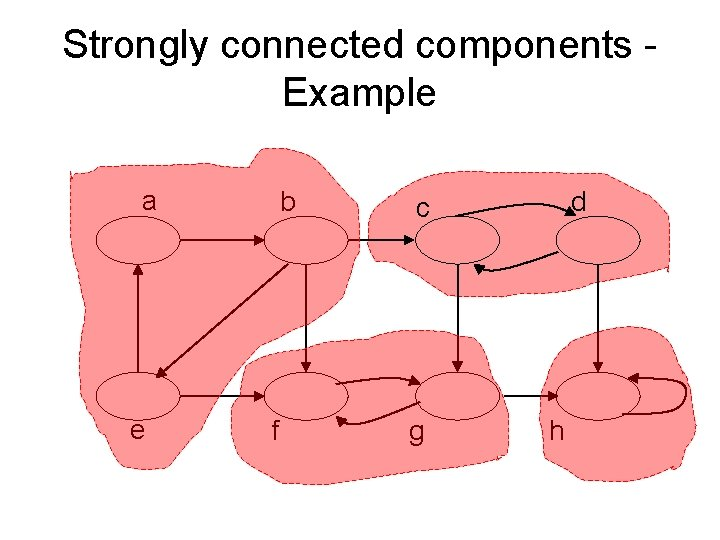Strongly connected components - Example a e b f d c g h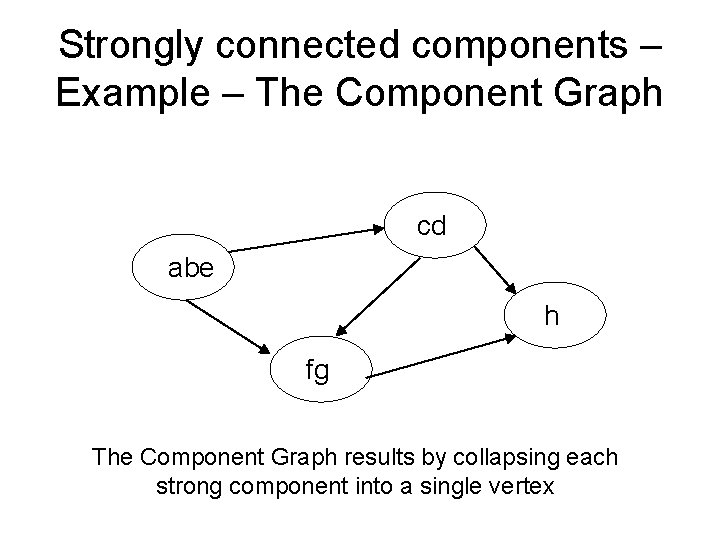Strongly connected components – Example – The Component Graph cd abe h fg The Component Graph results by collapsing each strong component into a single vertexThe Component Graph • Property: The Component Graph is a DAG (directed acyclic graph)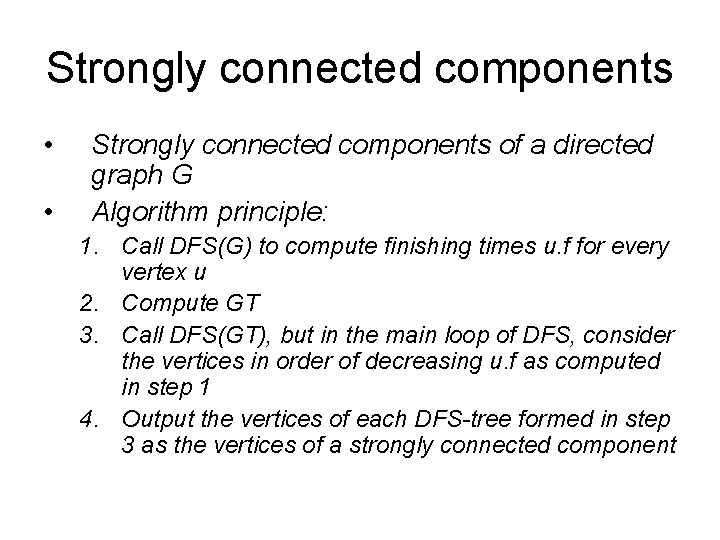Strongly connected components • • Strongly connected components of a directed graph G Algorithm principle: 1. Call DFS(G) to compute finishing times u. f for every vertex u 2. Compute GT 3. Call DFS(GT), but in the main loop of DFS, consider the vertices in order of decreasing u. f as computed in step 1 4. Output the vertices of each DFS-tree formed in step 3 as the vertices of a strongly connected component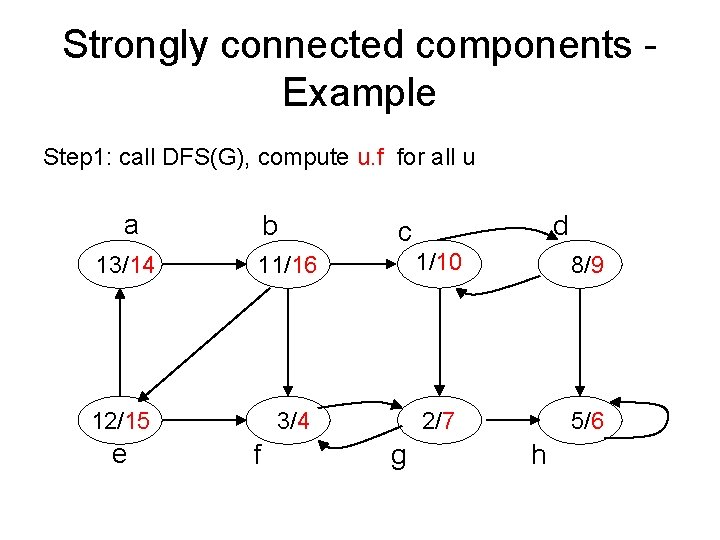Strongly connected components - Example Step 1: call DFS(G), compute u. f for all u a b d c 13/14 11/16 1/10 8/9 12/15 3/4 2/7 5/6 e f g h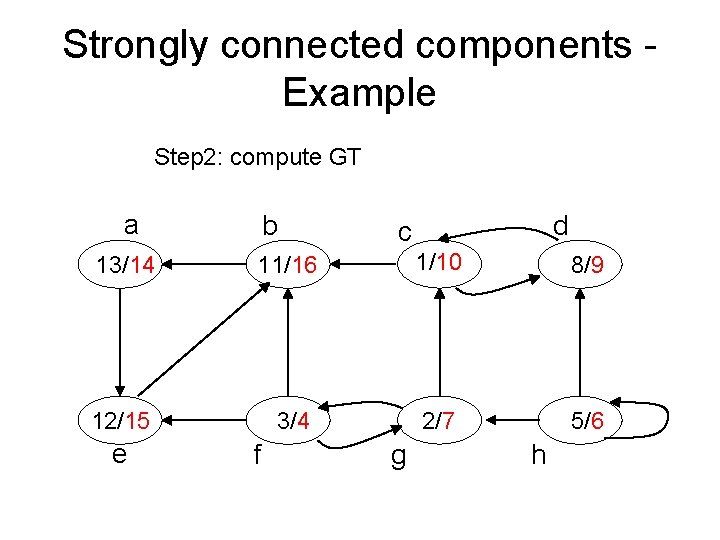Strongly connected components - Example Step 2: compute GT a b d c 13/14 11/16 1/10 8/9 12/15 3/4 2/7 5/6 e f g hStrongly connected components - Example Step 3: call DFS(GT), consider vertices in order of decreasing u. f a b d c 13/14 11/16 1/10 8/9 12/15 3/4 2/7 5/6 e f g hStrongly connected components - Example Step 3: call DFS(GT), consider vertices in order of decreasing u. f a b d c 13/14 11/16 1/10 8/9 12/15 3/4 2/7 5/6 e f g h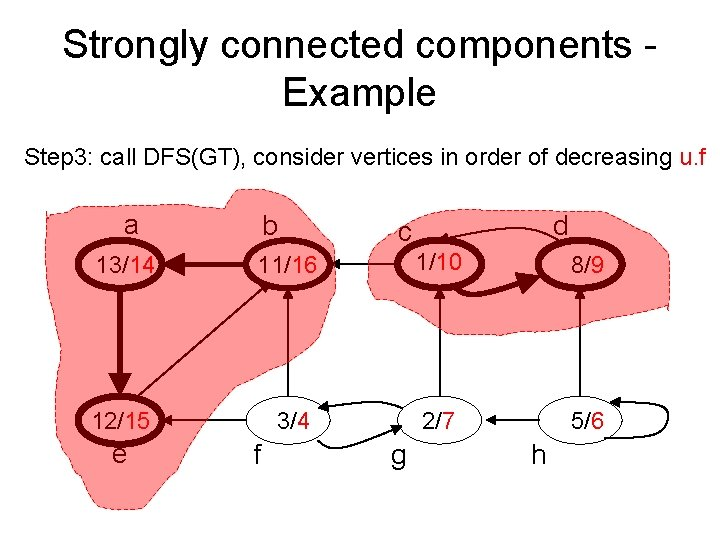Strongly connected components - Example Step 3: call DFS(GT), consider vertices in order of decreasing u. f a b d c 13/14 11/16 1/10 8/9 12/15 3/4 2/7 5/6 e f g hStrongly connected components - Example Step 3: call DFS(GT), consider vertices in order of decreasing u. f a b d c 13/14 11/16 1/10 8/9 12/15 3/4 2/7 5/6 e f g h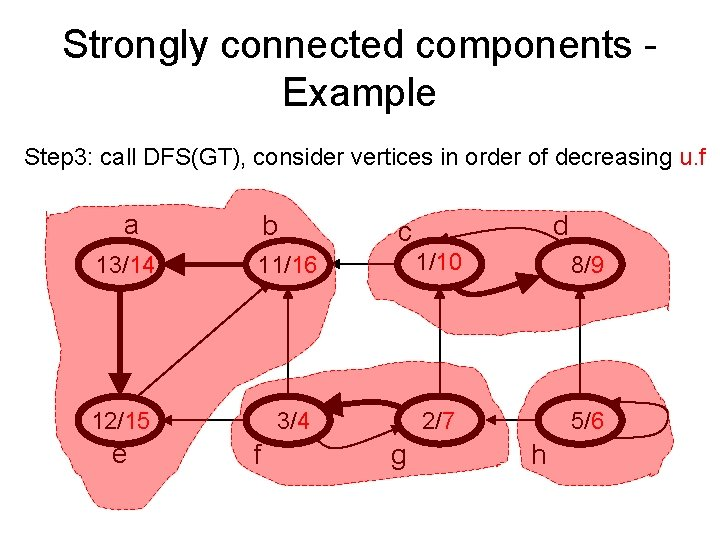Strongly connected components - Example Step 3: call DFS(GT), consider vertices in order of decreasing u. f a b d c 13/14 11/16 1/10 8/9 12/15 3/4 2/7 5/6 e f g hProof of Strong Components Algorithm • Theorem The Algorithm presented before finds the strong components of G. • Proof We must show that a set of vertices forms a strong components if and only if they are vertices of a tree in the DFS-forest of GT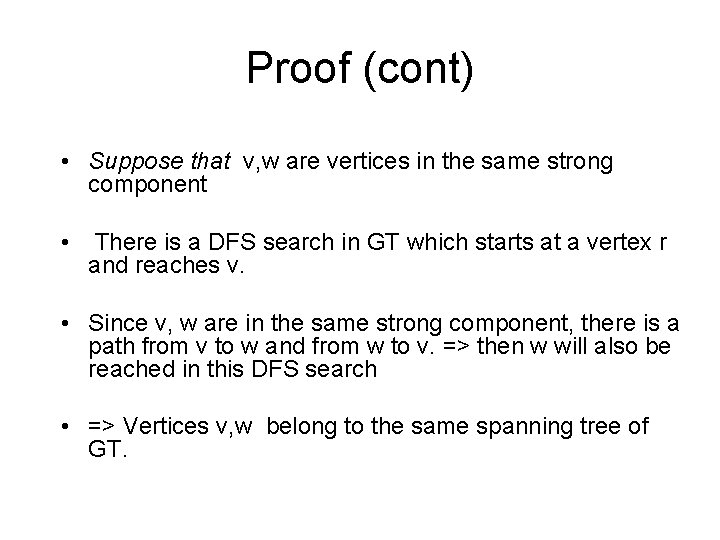Proof (cont) • Suppose that v, w are vertices in the same strong component • There is a DFS search in GT which starts at a vertex r and reaches v. • Since v, w are in the same strong component, there is a path from v to w and from w to v. => then w will also be reached in this DFS search • => Vertices v, w belong to the same spanning tree of GT.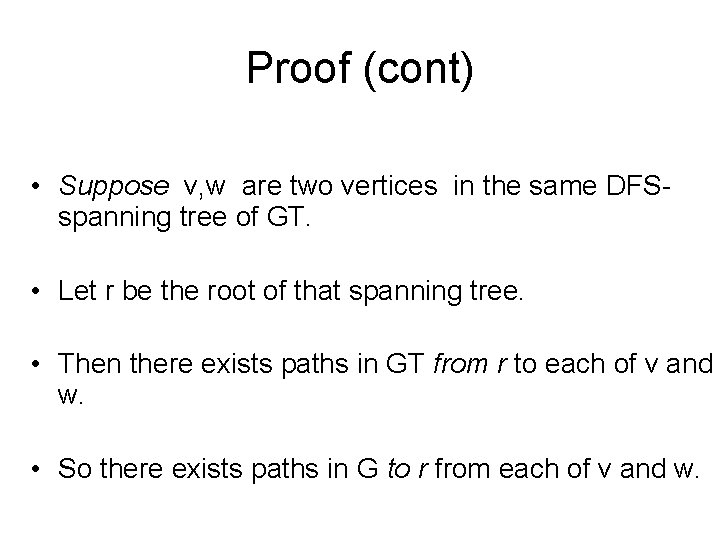Proof (cont) • Suppose v, w are two vertices in the same DFSspanning tree of GT. • Let r be the root of that spanning tree. • Then there exists paths in GT from r to each of v and w. • So there exists paths in G to r from each of v and w.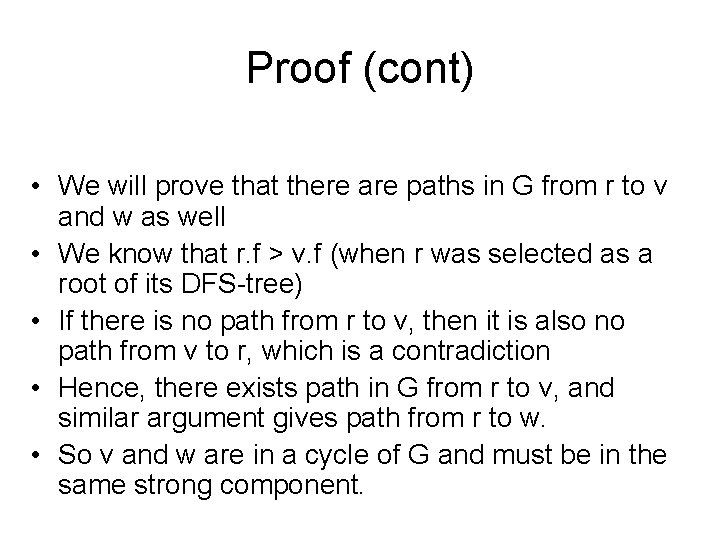Proof (cont) • We will prove that there are paths in G from r to v and w as well • We know that r. f > v. f (when r was selected as a root of its DFS-tree) • If there is no path from r to v, then it is also no path from v to r, which is a contradiction • Hence, there exists path in G from r to v, and similar argument gives path from r to w. • So v and w are in a cycle of G and must be in the same strong component.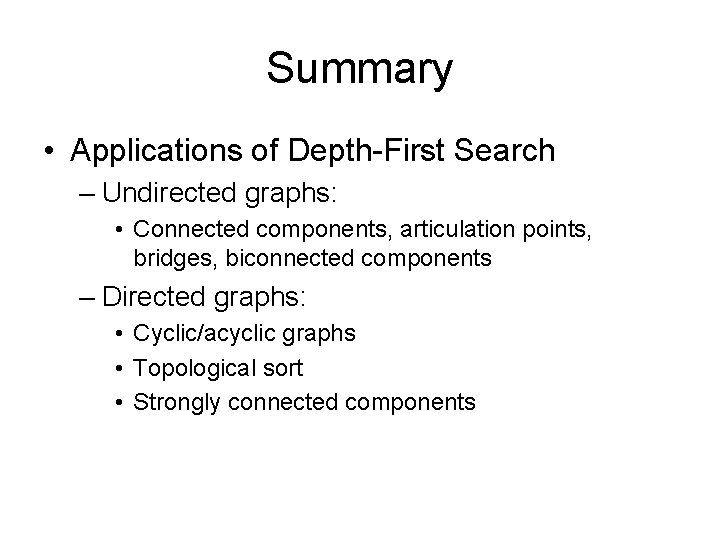Summary • Applications of Depth-First Search – Undirected graphs: • Connected components, articulation points, bridges, biconnected components – Directed graphs: • Cyclic/acyclic graphs • Topological sort • Strongly connected components